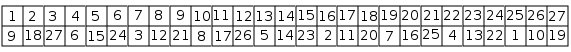# CAIA surprised me Part II The symmetries

For the cube problem described in the preceding post, CAIA begins with looking for symmetries in the problem formulation. What is a symmetry? For Richard Feynman: « A thing is symmetrical if there is something we can do to it so that after we have done it, it looks the same as it did before. »

There are many kinds of symmetries. CAIA only tries to find one of them: a permutation of the unknowns leads to the same set of constraints when each unknown is replaced by the corresponding one.

To do this, I defined a combinatorial problem: finding all the permutations of the unknowns that satisfy the preceding condition. This problem is given in the formalism used for all the combinatorial problems solved by CAIA. The interest of the bootstrap clearly appears here: knowing how to solve combinatorial problems allows us to solve problems necessary for solving combinatorial problems. For every new problem, CAIA solves a preliminary problem: finding which symmetries exist. Its data are the initial constraints of the main problem.

We are not restricted to the case where each constraint C is transformed in itself by the permutation, such as when one permutes A and B in the constraint A+B>0. Usually, most constraints are transformed into other ones.

For the cube problem, CAIA finds 47 solutions: for each solution that it finds, it can immediately add 47 new solutions.

One of these solutions is the following permutation:All the classical geometrical symmetries are among these 47 solutions: to the center, to the mean planes, etc. However, it found them from the constraints: it does not know geometry; the method can be used for problems where there is no geometrical interpretation. For instance, if unknowns A and B appear only in the constraint A+B=1,000,000 exchanging A and B gives the same constraint. Naturally, CAIA can often add new constraints for avoiding to generate a solution and its symmetrical ones. In the preceding example, if the values of A and B are different, adding constraint A<B will remove all the symmetrical solutions.

For three main reasons, CAIA needs finding symmetries:

1. This decreases the size of the search space, because constraints are added to prevent finding symmetrical solutions.

2. This increases the speed of the resolution process: for every new solution, one can immediately generate all its symmetrical solutions. For the cube, the system is 48 times faster once it has found the symmetries.

3. It is easier to create constraints: for each new constraint, CAIA adds all the constraints that are obtained by symmetry. Let us notice that it does not generate 47 other constraints for each constraint: as few unknowns are in a constraint, many of the constraints generated in that way are identical.

If one believes to help the system by adding constraints to the initial formulation, this is a mistake: this may hide some symmetries. For instance, one may be proud to have proven the constraint F(17)+F(11)=F(5)+F(23). Unfortunately, adding this constraint in the initial formulation will remove most of the symmetries, including the one given above. Indeed, the constraint that would be created with this symmetry is F(11)+F(17)=F(15)+F(13); it is not among the initial constraints of this new formulation. As it often happens, when one has a clever system, it is not good to try to help it: one may complicate its task. Let us trust CAIA to do its job.

Finally, for me, the main interest of finding symmetries is to show concretely that bootstrapping is possible, and can be very useful: realizing a solver for problems defined by a set of constraints improves the solver itself.

In the next post, we will see how CAIA uses these symmetries for solving the magic cube problem.